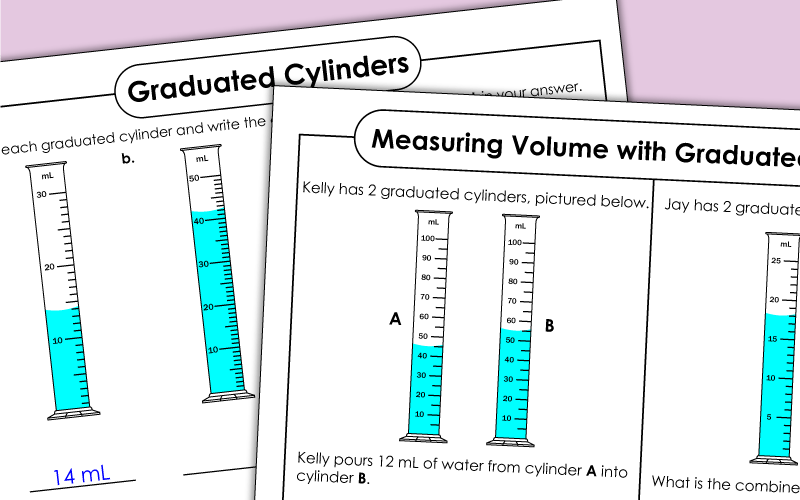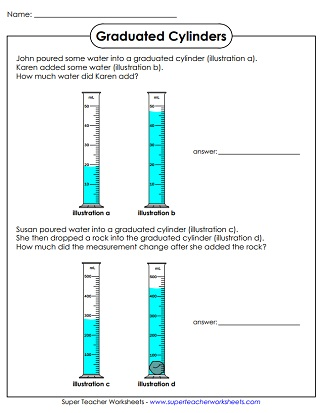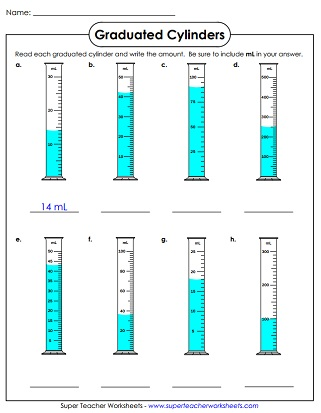Students must accurately read the measurements on the graduated cylinder illustrations. Includes scales that count by 1s, 5s, 10s, 20s, and 100s.## Intermediate - No Decimals

These mixed-scale cylinders do not feature decimal numbers.
Write the amount of liquid shown in each graduated cylinder.  The scale counts by 5s.
Look closely at each graduated cylinder picture and write the volume of water each contains.  This worksheet includes graduated cylinders that count by 10s.
How many milliliters of water is shown in each cylinder?  The scale counts by 100s.
Use subtraction to calculate the increase or decrease of liquids in the graduated cylinders.
Add, divide, and/or subtract to find the volumes of these graduated cylinders.

The scale of these graduated cylinders counts by 1s, which means students will need to use a decimal answer.
These graduated cylinders feature mixed scales, including 100s, 10s, 5s, and 1s.  Some answers will be a decimal number.

## Also on Super Teacher Worksheets...

Metric Capacity Worksheets

These worksheet feature capacity measurements in milliliters and liters.

Measurement Worksheets

Visit our collection of worksheets for measuring volume, length, and mass of objects.

## Sample Worksheet ImagesMy Account
Site Information